Home  │ Audio Home Page

Updated January 6, 2011.  See Document History at end for details.
Computer verified.

# Transfer Curve Shape and Distortion

### Introduction

Investigated here is the common belief that the more bends a transfer function has the worse the quality of its distortion.

### Mathematical Analysis

To evaluate the assertion at hand it is necessary to choose suitable mathematical models and methods.  I chose to use sin/cos functions as error functions added to a consistant linear function base (i.e. y = x - k(sin or cos)(m·x) ) in order to have the same sort of distortion characteristic regardless of the number of bends.  I also decided to iterate for a value of k in each case to give a total distortion of 0.01% to add another element of consistency.  The transfer curve graphs are exaggerated as a result because the actual transfer curves would still look very flat.  The methodology is to apply the transfer functions to one cycle of a unity magnitude sine function then analyze the result with a Fast Fourier Transform.  The resulting Fourier series analysis gives the distortion breakdown by harmonic.

Figure 1:  Transfer Curve 1   y = x - k·cos(0.5π·x)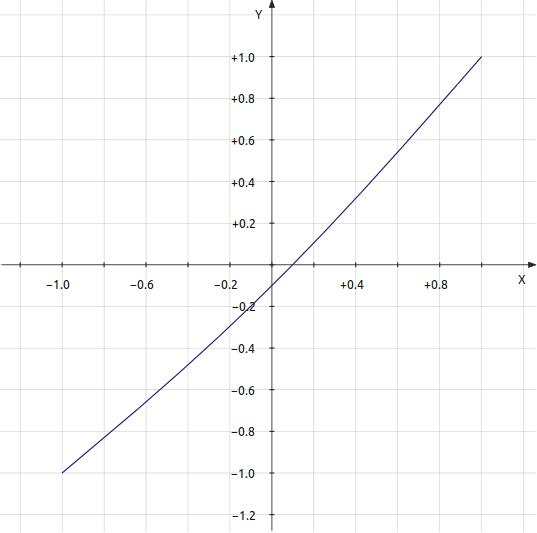k = 0.000189781
Total distortion = 0.0001002042  0.01002042%    -79.9823dB
Breakdown by harmonic
 harmonic # value decibels 1 1.0000000000 0.0000dB 2 0.0000947773 -80.4659dB 4 0.0000053124 -105.4942dB 6 0.0000001132 -138.9199dB 8 0.0000000013 -177.9077dB

Figure 2:  Transfer Curve 2
y = x - k·sin(1.0π·x)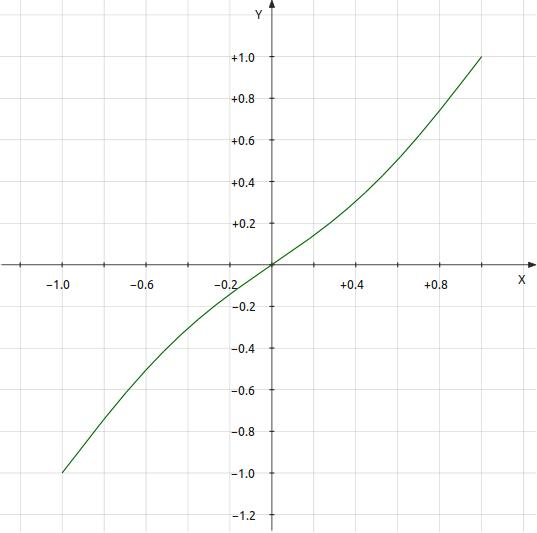k = 0.000128746
Total distortion = 0.0001002025  0.01002025%    -79.9824dB
Breakdown by harmonic
 harmonic # value decibels 1 0.9999267138 -0.0006dB 3 0.0000858629 -81.3239dB 5 0.0000134259 -97.4411dB 7 0.0000008807 -121.1034dB 9 0.0000000322 -149.8463dB 11 0.0000000008 -182.4618dB

Figure 3:  Transfer Curve 3
y = x - k·cos(1.5π·x)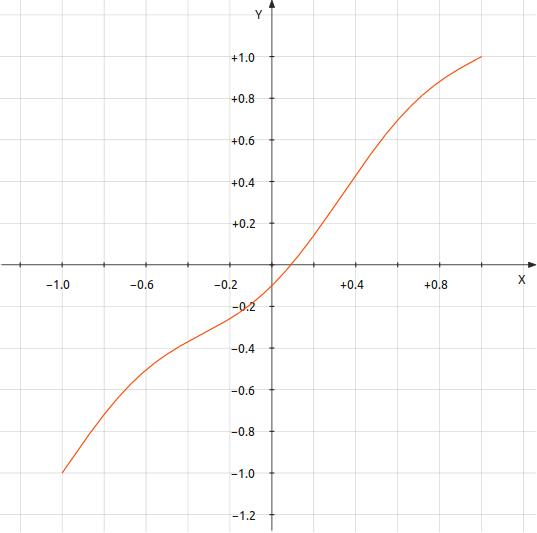k = 7.9155e-005
Total distortion = 0.0001001989  0.01001989%    -79.9827dB
Breakdown by harmonic
 harmonic # value decibels 1 1.0000000000 0.0000dB 2 0.0000231636 -92.7039dB 4 0.0000586435 -84.6356dB 6 0.0000162764 -95.7689dB 8 0.0000019717 -114.1033dB 10 0.0000001373 -137.2439dB 12 0.0000000063 -164.0541dB 14 0.0000000002 -193.8519dB

Figure 4:  Transfer Curve 4
y = x - k·sin(2.0π·x)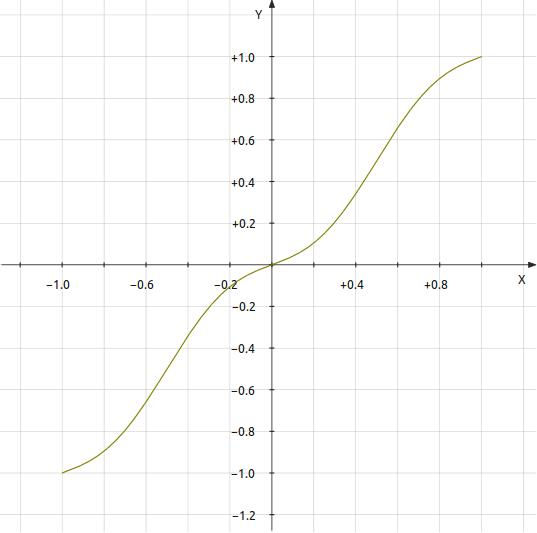k = 8.4877e-005
Total distortion = 0.0001004889  0.01004889%    -79.9576dB
Breakdown by harmonic
 harmonic # value decibels 1 1.0000360528 0.0003dB 3 0.0000049419 -106.1221dB 5 0.0000632885 -83.9735dB 7 0.0000267398 -91.4568dB 9 0.0000049439 -106.1186dB 11 0.0000005344 -125.4434dB 13 0.0000000384 -148.3066dB 15 0.0000000020 -174.0485dB

Figure 5:  Transfer Curve 5
y = x - k·cos(2.5π·x)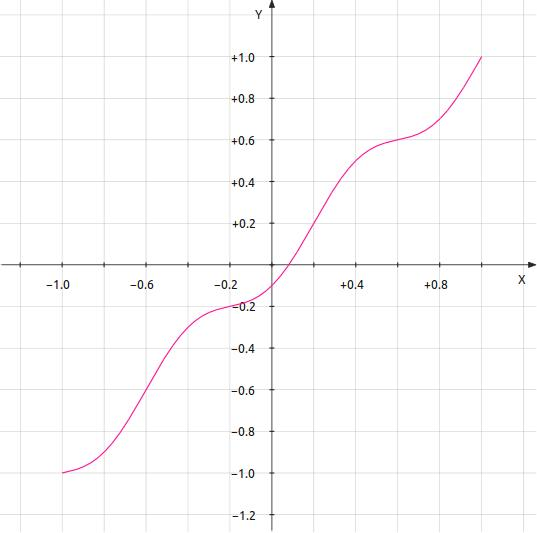k = 6.00815e-005
Total distortion = 0.0001006654  0.01006654%    -79.9424dB
Breakdown by harmonic
 harmonic# value decibels 1 1.0000000000 0.0000dB 2 0.0000180809 -94.8556dB 4 0.0000083474 -101.5690dB 6 0.0000415688 -87.6247dB 8 0.0000251344 -91.9946dB 10 0.0000064539 -103.8036dB 12 0.0000009738 -120.2309dB 14 0.0000000986 -140.1187dB 16 0.0000000072 -162.8095dB 18 0.0000000004 -187.8707dB

You should not read too much into the fact the the harmonic results are entirely even or odd.  This is a function of the  perfect symmetry of the trigonometric error functions used.  However there is a trend here.  The more bends in the transfer function, the higher the order of the highest harmonics that result.

### Topology Considerations

Care must be taken if successive stages of a system are not to create multiple bends in the transfer curve.  It is commonly thought that bending the transfer function in an opposite directions alternatively will reduce distortion.  And it may.  However the nature of most transfer functions prevents distortion cancellation.  Instead the flatter curve will have more bends.  This is because the transfer curves of known amplifying devices have a slope that increases with magnitude, resulting in the greatest bend near cutoff.  Alternate bends under varying gain and bias contexts will create random bends to a less musical end.  A common topology creating an opportunity for multiple bends is one where the same polarity of device is used in successsive inverting stages (See figure 6 below).  Vacuum tube circuits force this type of topology in some cases because tubes have only n-type transfer curves.  Perhaps this is why SET fans scorn multiple amplifiying stages in their signal paths, sometimes only putting a passive volume control in front of their single-stage amp.  Transistors have an advantage here because n-type and p-type devices are available enabling an alternating topology to match the bends in the transfer curves in the same direction relative to the signal (See figure 7 below).
 Figure 6:  cascaded n-channel topology can create as many bends in the transfer curve as inverting stages.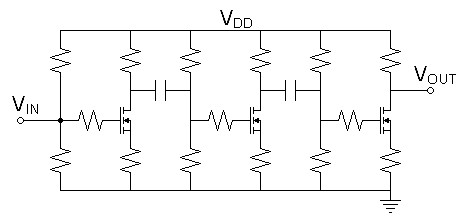Figure 7:  Alternating topology bends the transfer curve in the same direction relative to the signal in each device.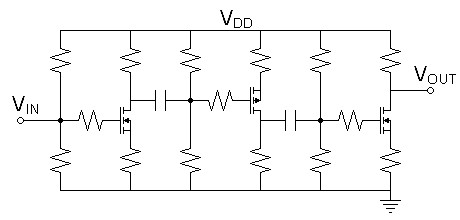Perhaps one might think to overcome transfer function anomalies by having one characteristic or bend in a transfer function dominate the others.  Will the dominant bend solely determine the distortion outcome?  First I added the error term of transfer curve 5 reduced by 10 to transfer curve 1 then determined this an improper model.  The proper model below nests the transfer functions instead, a more proper representation.  The result is somewhat inconclusive.  Relative to multibend transfer curve, the second harmonic is raised, the fourth lowered, odds are added at low levels, the remainder lower.  The result appears mostly additive with some subtractions due to phase.  It appears the intended masking that results would be less than ideal.  This might infer that using a euphonic stage to mask the distortion of a less than euphonic signal chain would require that chain to already have inaudible distortion.

Figure 8:  Transfer Curve 6   y = x - 0.1k·cos(2.5π·x) - k·cos(0.5π·(x - 0.1k·cos(2.5π·x)))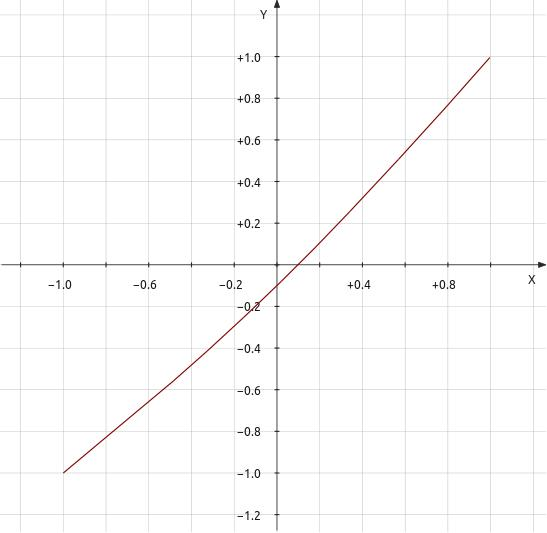k = 0.000164986
Total distortion = 0.0001002453  0.01002453%    -79.9787dB
Breakdown by harmonic
 harmonic# value decibels dominant bend (from figure 1) full multibend (from figure 5) 1 0.9999999983 -0.0000dB -0.0000dB 0.0000dB 2 0.0000774293 -82.2219dB -80.4659dB -94.8556dB 3 0.0000000005 -186.3172dB 4 0.0000023261 -112.6675dB -105.4942dB -101.5690dB 5 0.0000000022 -173.0612dB 6 0.0000115134 -98.7760dB -138.9199dB -87.6247dB 7 0.0000000006 -184.6238dB 8 0.0000069031 -103.2191dB -177.9077dB -91.9946dB 9 0.0000000010 -180.4310dB 10 0.0000017723 -115.0295dB -103.8036dB 11 0.0000000004 -189.1018dB 12 0.0000002674 -131.4568dB -120.2309dB 14 0.0000000271 -151.3446dB -140.1187dB 16 0.0000000020 -174.0354dB -162.8095dB 18 0.0000000001 -199.0969dB -187.8707dB

### Final Thoughts

Investigating the use of distortion cancellation in some topologies may add additional benefit to this pursuit.  Other than a matched current mirror, I cannot immediately think of any circuit for which this would be an easy task.  The expensive OPA627 operational amplifier derived its reputation for low distortion and quality sound from a proprietary distortion cancelling circuit.

Document History
January 1, 2011  Created.
January 1, 2011  Made minor grammatical corrections.